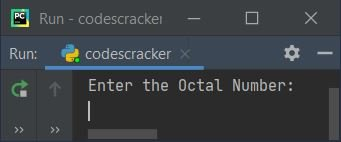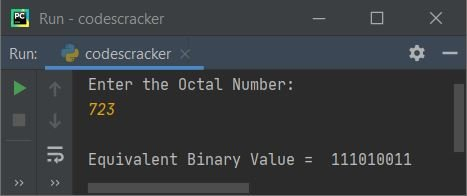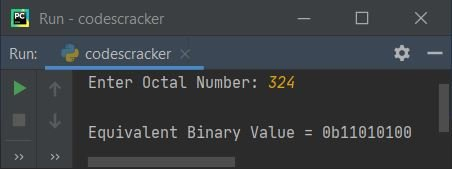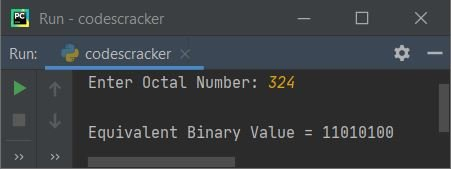# Python Program to Convert Octal to Binary

In this article, I've created some programs in Python, to convert octal number entered by user to its equivalent binary value. Here are the list of programs:

• Octal to Binary using while Loop
• Using int() and bin() Methods
• Using Function
• Using Class

Note - Before creating these programs, if you're not aware about steps used for the conversion, then refer to Octal to Binary Formula and Example to get every required things.

## Octal to Binary using while Loop

To convert octal to binary number in Python, you have to ask from user to enter any octal number, then convert that number into its binary equivalent. The question is, write a Python program to convert octal number to binary using while loop. Here is its answer:

```print("Enter the Octal Number: ")
octnum = int(input())

rev = 0
chk = 0

while octnum!=0:
rem = octnum%10
if rem>7:
chk = 1
break
rev = rem + (rev*10)
octnum = int(octnum/10)

if chk == 0:
octnum = rev
binnum = ""

while octnum!=0:
rem = octnum%10
if rem==0:
binnum = binnum + "000"
elif rem==1:
binnum = binnum + "001"
elif rem==2:
binnum = binnum + "010"
elif rem==3:
binnum = binnum + "011"
elif rem==4:
binnum = binnum + "100"
elif rem==5:
binnum = binnum + "101"
elif rem==6:
binnum = binnum + "110"
elif rem==7:
binnum = binnum + "111"
octnum = int(octnum/10)

print("\nEquivalent Binary Value = ", binnum)

else:
print("\nInvalid Input!")```

Here is its sample run:Now supply the input say 723 as octal number and press `ENTER` key to convert and print its equivalent binary value as shown in the snapshot given below:The dry run of above program with user input 723 goes like:

• Initial values, octnum=723 (entered by user), rev=0, chk=0
• Now the condition (of while loop) octnum!=0 or 723!=0 evaluates to be true, therefore program flow goes inside the loop. This loop is created to reverse the entered octal number. Because, we've to convert binary equivalent of each and every digit. But using rem = octnum%10 statement, the digit we get is the last digit at first time. Therefore we've reversed the number before conversion
• Inside the loop, octnum%10 or 723%10 or 3 gets initialized to rem. So rem=3
• Now the condition (of if) rem>7 or 3>7 evaluates to be false, therefore the program flow does not goes inside this if's body. Here we've checked whether any digit is greater than 7 or not. Because 8, 9 are both invalid octal digits
• Now rem + (rev*10) or 3 + (0*10) or 3 gets initialized to rev
• int(octnum/10) or int(723/10) or 72 gets initialized to octnum
• Program flow goes back and evaluates the condition of while loop again with new value of octnum.
• That is, the condition octnum!=0 or 72!=0 evaluates to be true again, therefore program flow again goes inside the loop. This process continues until the condition evaluates to be false
• In this way, after exiting from the loop when its condition evaluates to be false, the variable rev holds its value as 327. Therefore rev=327
• Now the condition chk==0 or 0==0 evaluates to be true, therefore program flow goes inside the loop
• rev or 327 gets initialized to octnum. And "" gets initialized to binnum. That is, a string variable binnum with empty content gets defined
• Now the condition (of inner while loop) octnum!=0 or 327!=0 evaluates to be true, therefore program flow goes inside the loop
• And octnum%10 or 327%10 or 7 gets initialized to rem
• Since the value of rem is 7, therefore the condition of last elif, that is rem==7 or 7==7 evaluates to be true, therefore binnum + "111" or "" + "111" or "111" gets initialized to binnum
• Then int(octnum/10) or int(327/10 or 32 gets initialized to octnum
• Now program flow goes back and evaluates the condition of while loop (inner) again
• That is, the condition octnum!=0 or 32!=0 evaluates to be true again, therefore program flow goes inside the loop again. This process continues until the condition evaluates to be false
• In this way, the given octal value gets converted into its equivalent binary value
• Now print the value of binnum after exiting from the loop

## Octal to Binary using int() and bin()

This program uses int() and bin(), predefined methods of Python to do the same job as of previous program creating using complete user-defined code. The int() is used to convert a value passed as its argument to its integer equivalent. Whereas bin() returns binary equivalent of value passed as its argument.

```print("Enter Octal Number: ", end="")
onum = input()

bnum = int(onum, 8)
bnum = bin(bnum)

print("\nEquivalent Binary Value =", bnum)```

Here is its sample run with user input 324:Note - To skip first two characters, add [2:] just after bnum while printing. That is, replace the following statement:

`print("\nEquivalent Binary Value =", bnum)`

with the statement given below:

`print("\nEquivalent Binary Value =", bnum[2:])`

Now the output looks like, with same user input as of previous sample run:Note - The end= is used to skip printing of an automatic newline using print()

## Octal to Binary using Function

This program uses user-defined function named OctToBin() to convert octal to binary. The function takes a number (octal) as its argument and returns its equivalent binary value using int() and bin():

```def OctToBin(o):
return bin(int(o, 8))

print("Enter Octal Number: ", end="")
onum = input()

bnum = OctToBin(onum)
print("\nEquivalent Binary Value =", bnum[2:])```

## Octal to Binary using Class

This is the last program of this article, created using a class named CodesCracker. Class is an object-oriented feature of Python.

```class CodesCracker:
def OctToBin(self, o):
return bin(int(o, 8))

print("Enter Octal Number: ", end="")
onum = input()

obj = CodesCracker()
bnum = obj.OctToBin(onum)
print("\nEquivalent Binary Value =", bnum[2:])```

An object obj of class CodesCracker is created to access its member function, OctToBin() using dot (.) operator.

#### Same Program in Other Languages

Python Online Test

« Previous Program Next Program »